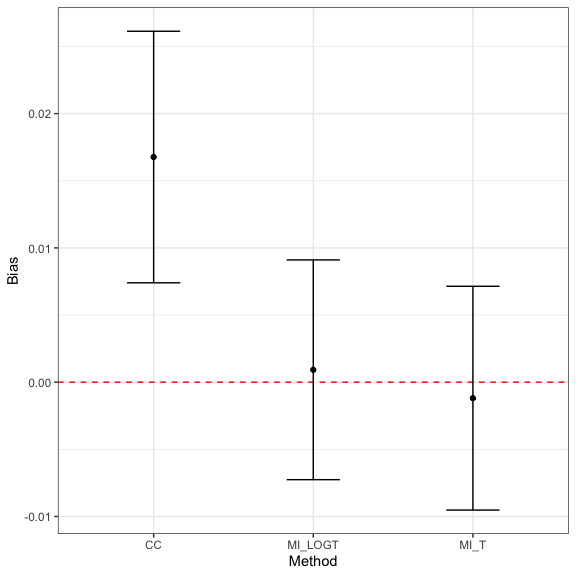# rsimsum and the tidyverse

#### 2019-09-12

rsimsum plays nice with the tidyverse.

library(rsimsum)
library(dplyr)
#>
#> Attaching package: 'dplyr'
#> The following objects are masked from 'package:stats':
#>
#>     filter, lag
#> The following objects are masked from 'package:base':
#>
#>     intersect, setdiff, setequal, union
library(ggplot2)
library(knitr)

For instance, it is possible to chain functions using the piping operator %>% to obtain tables and plots with a single call:

data("MIsim", package = "rsimsum")
MIsim %>%
simsum(estvarname = "b", se = "se", methodvar = "method", true = 0.5) %>%
summary() %>%
get_data() %>%
kable()
#> 'ref' method was not specified, CC set as the reference
stat est mcse method lower upper
nsim 1000.0000000 NA CC NA NA
thetamean 0.5167662 NA CC NA NA
thetamedian 0.5069935 NA CC NA NA
se2mean 0.0216373 NA CC NA NA
se2median 0.0211425 NA CC NA NA
bias 0.0167662 0.0047787 CC 0.0074001 0.0261322
empse 0.1511150 0.0033807 CC 0.1444889 0.1577411
mse 0.0230940 0.0011338 CC 0.0208717 0.0253163
relprec 0.0000000 0.0000000 CC 0.0000000 0.0000000
modelse 0.1470963 0.0005274 CC 0.1460626 0.1481300
relerror -2.6593842 2.2049438 CC -6.9809947 1.6622263
cover 0.9430000 0.0073315 CC 0.9286305 0.9573695
becover 0.9400000 0.0075100 CC 0.9252807 0.9547193
power 0.9460000 0.0071473 CC 0.9319915 0.9600085
nsim 1000.0000000 NA MI_LOGT NA NA
thetamean 0.5009231 NA MI_LOGT NA NA
thetamedian 0.4969223 NA MI_LOGT NA NA
se2mean 0.0182091 NA MI_LOGT NA NA
se2median 0.0172157 NA MI_LOGT NA NA
bias 0.0009231 0.0041744 MI_LOGT -0.0072586 0.0091048
empse 0.1320064 0.0029532 MI_LOGT 0.1262182 0.1377947
mse 0.0174091 0.0008813 MI_LOGT 0.0156818 0.0191364
relprec 31.0463410 3.9374726 MI_LOGT 23.3290364 38.7636456
modelse 0.1349413 0.0006046 MI_LOGT 0.1337563 0.1361263
relerror 2.2232593 2.3317773 MI_LOGT -2.3469401 6.7934588
cover 0.9490000 0.0069569 MI_LOGT 0.9353647 0.9626353
becover 0.9490000 0.0069569 MI_LOGT 0.9353647 0.9626353
power 0.9690000 0.0054808 MI_LOGT 0.9582579 0.9797421
nsim 1000.0000000 NA MI_T NA NA
thetamean 0.4988092 NA MI_T NA NA
thetamedian 0.4939111 NA MI_T NA NA
se2mean 0.0179117 NA MI_T NA NA
se2median 0.0169319 NA MI_T NA NA
bias -0.0011908 0.0042510 MI_T -0.0095226 0.0071409
empse 0.1344277 0.0030074 MI_T 0.1285333 0.1403221
mse 0.0180542 0.0009112 MI_T 0.0162682 0.0198401
relprec 26.3681613 3.8423791 MI_T 18.8372366 33.8990859
modelse 0.1338346 0.0005856 MI_T 0.1326867 0.1349824
relerror -0.4412233 2.2689748 MI_T -4.8883321 4.0058856
cover 0.9430000 0.0073315 MI_T 0.9286305 0.9573695
becover 0.9430000 0.0073315 MI_T 0.9286305 0.9573695
power 0.9630000 0.0059692 MI_T 0.9513006 0.9746994
MIsim %>%
simsum(estvarname = "b", se = "se", methodvar = "method", true = 0.5) %>%
summary() %>%
get_data(stats = "bias") %>%
ggplot(aes(x = method, y = est, ymin = lower, ymax = upper)) +
geom_hline(yintercept = 0, color = "red", lty = "dashed") +
geom_point() +
geom_errorbar(width = 1 / 3) +
theme_bw() +
labs(x = "Method", y = "Bias")
#> 'ref' method was not specified, CC set as the reference